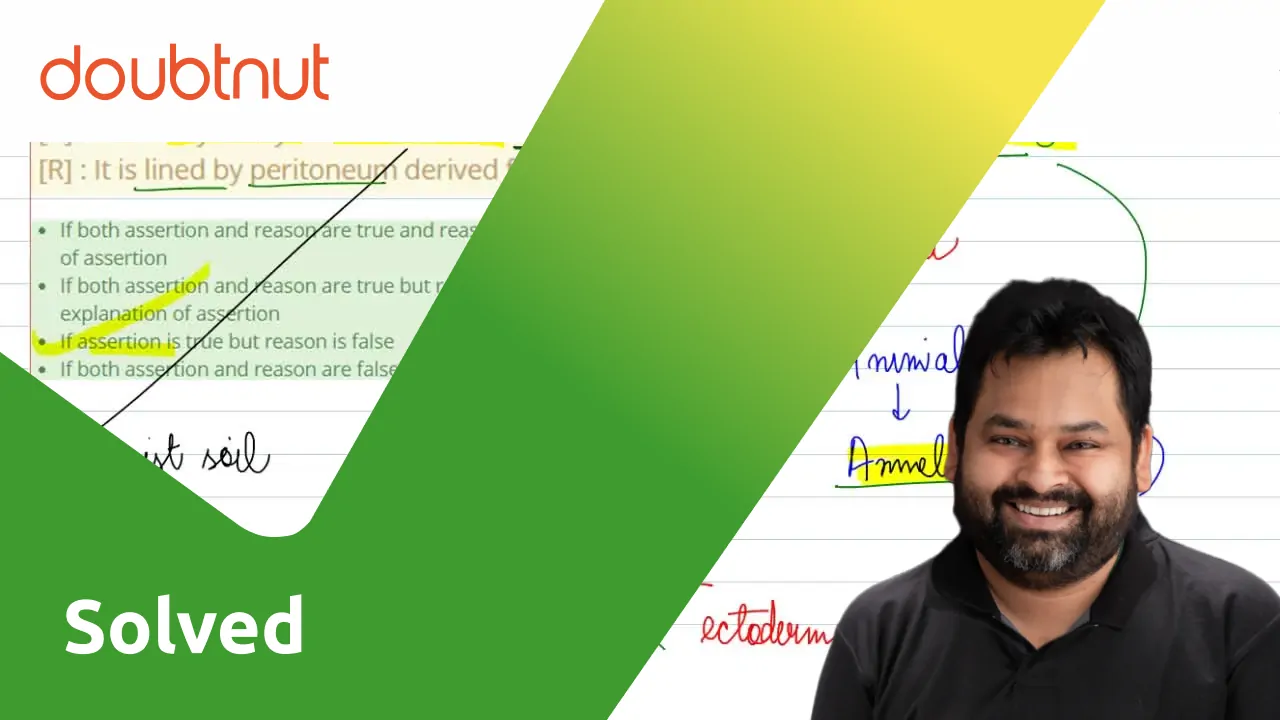Doubtnut App - Get 50 Lakh+ video solutions better than GoogleInstall Now

# [A] : The body cavity of earthworm is a true coelem . [R] : It is lined by peritoneum derived from ectoderm .

A

If both A and R are true and R is the correct explanation of A

B

If both A and R are true but R is not the correct explanation of A

C

If A is true and R is false

D

If both A and R are false

Video Solution